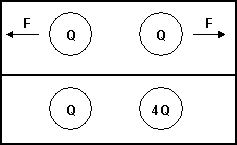Teaching Physics with the Physics SuiteEdward F. Redish

Forces between charges*

 Two small objects each with a net charge of Q (where Q is a positive number) exert a force of magnitude F on each other. We replace one of the objects with another whose net charge is 4Q.(i) The original magnitude of the force on the Q charge was F; what is the magnitude of the force on the Q now?

 (a) 16F (b) 4F (c) F (d) F/4 (e) other

(ii). What is the magnitude of the force on the 4Q charge?

 (a) 16F (b) 4F (c) F (d) F/4 (e) other

(iii) Next, we move the Q and 4Q charges to be 3 times as far apart as they were. Now what is the magnitude of the force on the 4Q?

 (a) F/9 (b) F/3 (c) 4F/9 (d) 4F/3 (e) other

(iv) In the original state (2 charges Q) if the symbol Q were taken to have a negative value, how would the forces change compared to the original state?

 (a) stay the same (b) both would reverse (c) left one would reverse (d) right one would reverse (e) none of the above.

* From the CSEM.

Not finding what you wanted? Check the Site Map for more information.